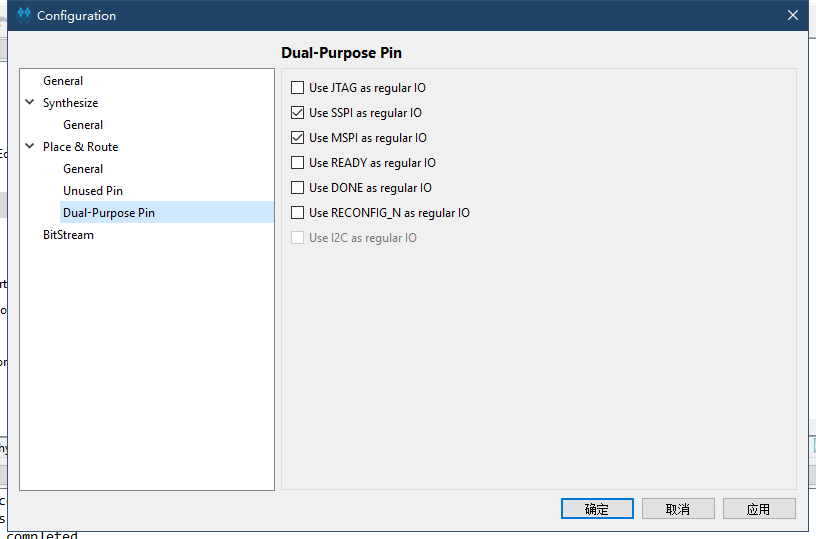# RGB LCD 示例

## 先介绍时序

RGB LCD 显示协议和 VGA 类似，通信都有专用的行同步、场同步信号线。它们的主要区别是前者传输用的是数字信号，后者传输走的是模拟信号。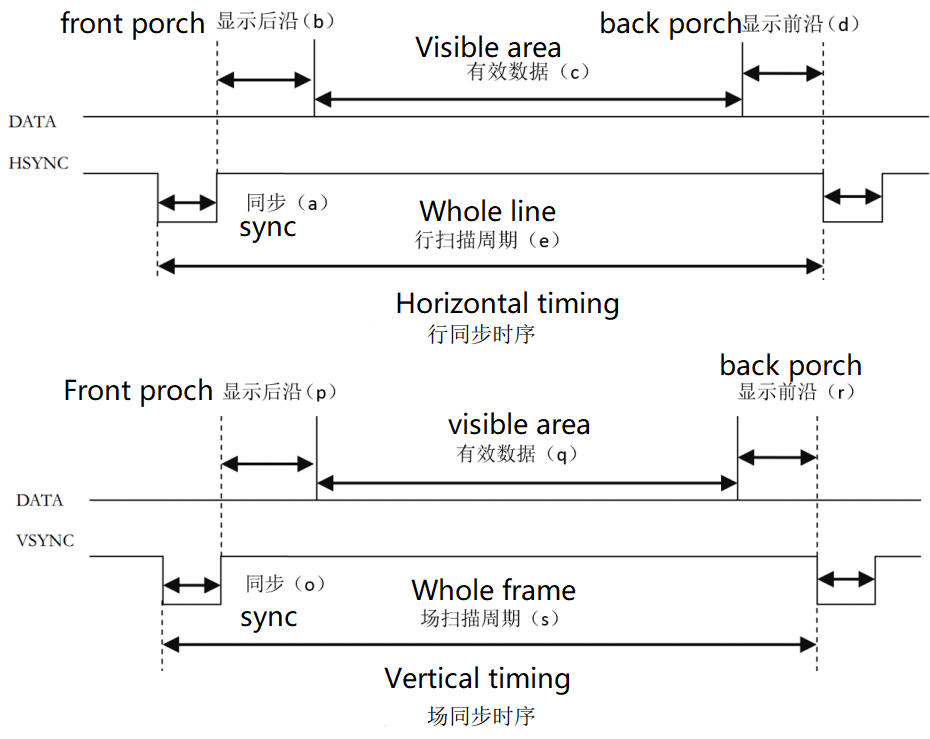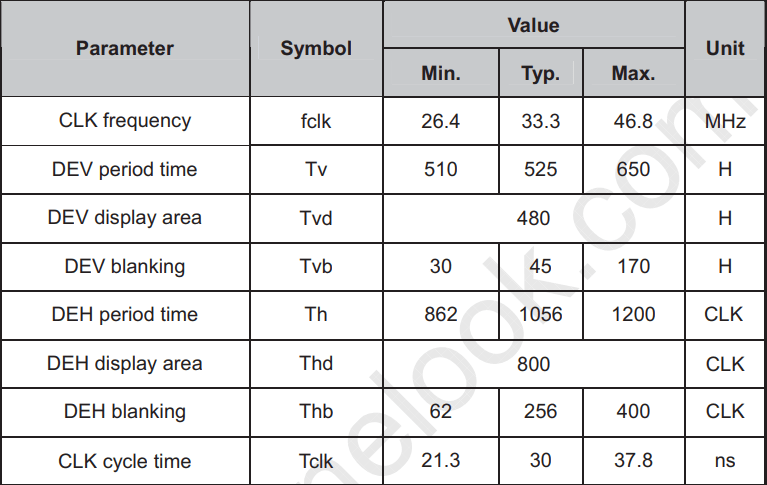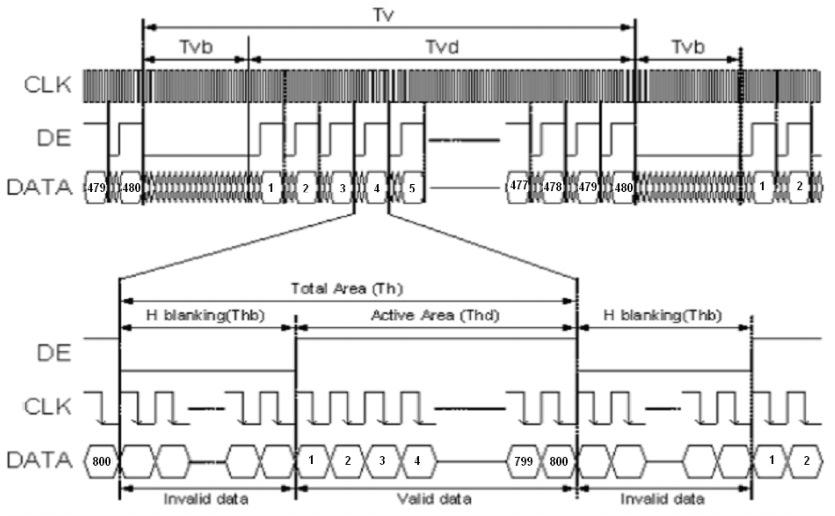## 生成屏幕时钟

• 这里需要用到高云半导体官方的IP核

### pll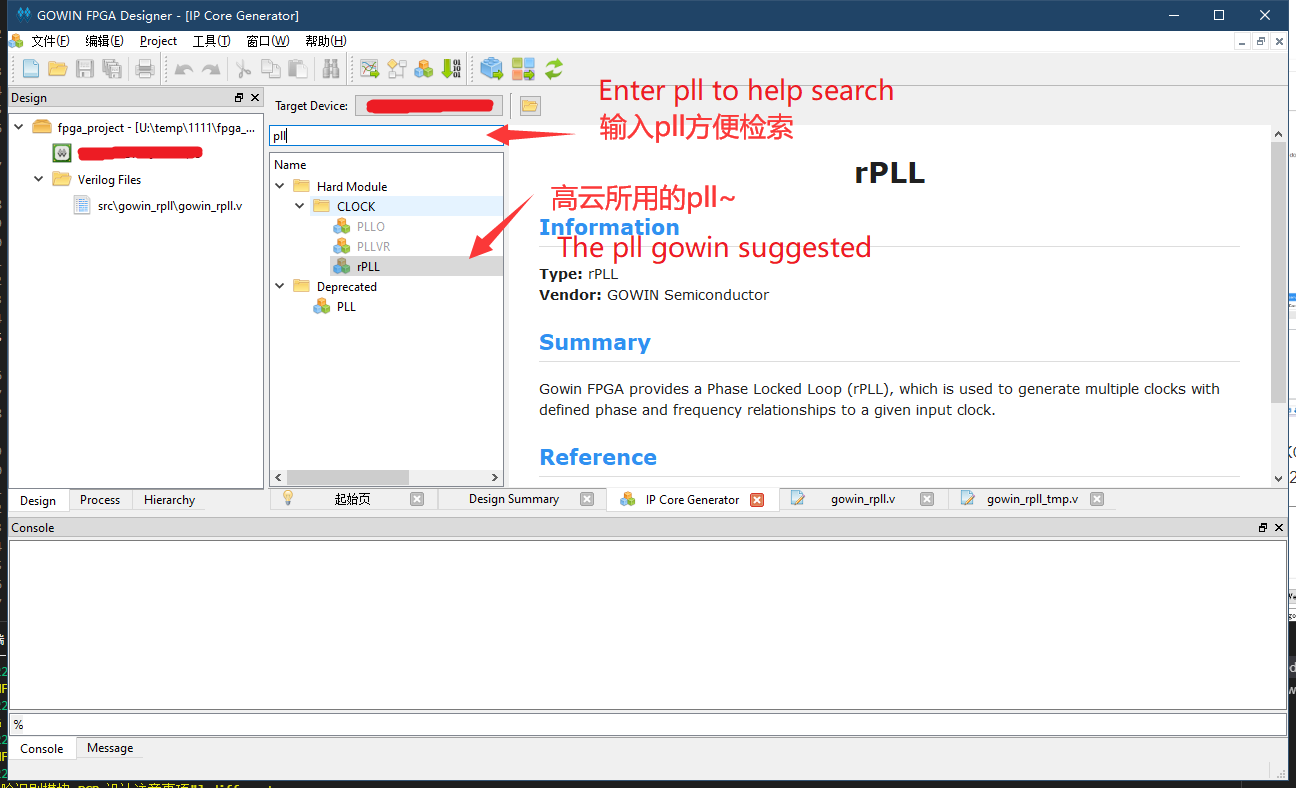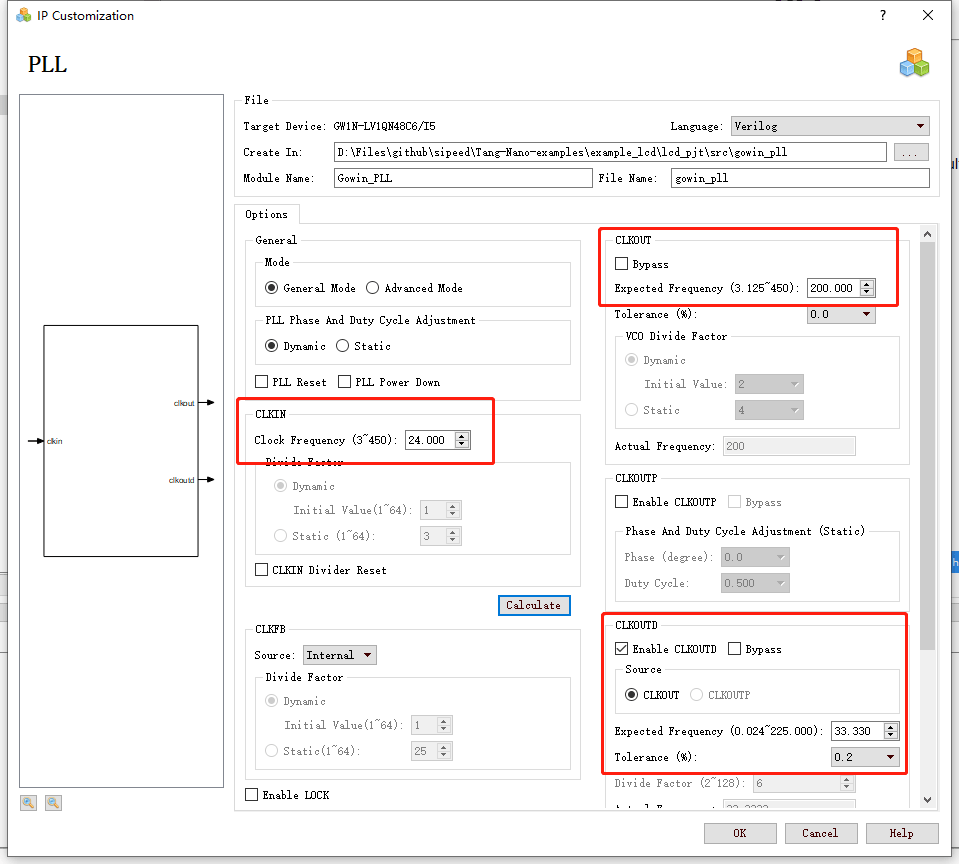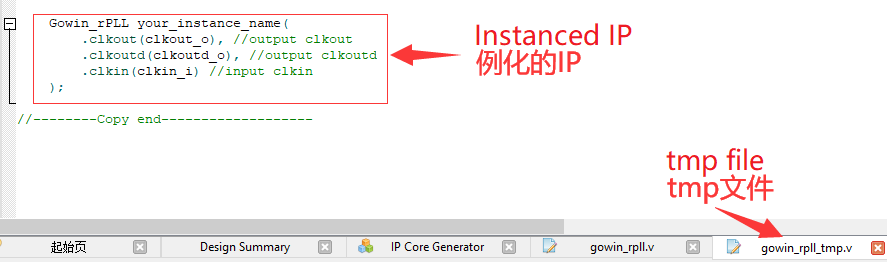## 屏幕驱动代码

• 首先新建一个额外的verilog文件来保存下面要编写的代码

### 端口定义

module VGAMod
(
input                   CLK,
input                   nRST,

input                   PixelClk,

output                  LCD_DE,
output                  LCD_HSYNC,
output                  LCD_VSYNC,

output          [4:0]   LCD_B,
output          [5:0]   LCD_G,
output          [4:0]   LCD_R
);


### 时序常量


localparam      V_BackPorch = 16'd6; //0 or 45
localparam      V_Pluse 	= 16'd5;
localparam      HightPixel  = 16'd480;
localparam      V_FrontPorch= 16'd62; //45 or 0

localparam      H_BackPorch = 16'd182;
localparam      H_Pluse 	= 16'd1;
localparam      WidthPixel  = 16'd800;
localparam      H_FrontPorch= 16'd210;

localparam      PixelForHS  =   WidthPixel + H_BackPorch + H_FrontPorch;
localparam      LineForVS   =   HightPixel + V_BackPorch + V_FrontPorch;



### 定义变量

• 定义一些变量能够容易编写程序

reg [15:0] LineCount;
reg [15:0] PixelCount;

reg	[9:0]  Data_R;
reg	[9:0]  Data_G;
reg	[9:0]  Data_B;



### 同步信号

    always @(  posedge PixelClk or negedge nRST  )begin
if( !nRST ) begin
LineCount       <=  16'b0;
PixelCount      <=  16'b0;
end
else if(  PixelCount  ==  PixelForHS ) begin
PixelCount      <=  16'b0;
LineCount       <=  LineCount + 1'b1;
end
else if(  LineCount  == LineForVS  ) begin
LineCount       <=  16'b0;
PixelCount      <=  16'b0;
end
else
PixelCount      <=  PixelCount + 1'b1;
end

always @(  posedge PixelClk or negedge nRST  )begin
if( !nRST ) begin
Data_R <= 9'b0;
Data_G <= 9'b0;
Data_B <= 9'b0;
end
else begin
end
end

//注意这里HSYNC和VSYNC负极性
assign  LCD_HSYNC = (( PixelCount >= H_Pluse)&&( PixelCount <= (PixelForHS-H_FrontPorch))) ? 1'b0 : 1'b1;
assign  LCD_VSYNC = ((( LineCount  >= V_Pluse )&&( LineCount  <= (LineForVS-0) )) ) ? 1'b0 : 1'b1;


### 使能信号

assign  LCD_DE = (  ( PixelCount >= H_BackPorch )&&
( PixelCount <= PixelForHS-H_FrontPorch ) &&
( LineCount >= V_BackPorch ) &&
( LineCount <= LineForVS-V_FrontPorch-1 ))  ? 1'b1 : 1'b0;
//这里不减一，会抖动


### 测试彩条

• 这段代码用来产生 LCD 的测试数据，产生彩条显示
    localparam          Colorbar_width   =   WidthPixel / 16;

assign  LCD_R     = ( PixelCount < ( H_BackPorch +  Colorbar_width * 0  )) ? 5'b00000 :
( PixelCount < ( H_BackPorch +  Colorbar_width * 1  )) ? 5'b00001 :
( PixelCount < ( H_BackPorch +  Colorbar_width * 2  )) ? 5'b00010 :
( PixelCount < ( H_BackPorch +  Colorbar_width * 3  )) ? 5'b00100 :
( PixelCount < ( H_BackPorch +  Colorbar_width * 4  )) ? 5'b01000 :
( PixelCount < ( H_BackPorch +  Colorbar_width * 5  )) ? 5'b10000 :  5'b00000;

assign  LCD_G    =  ( PixelCount < ( H_BackPorch +  Colorbar_width * 6  )) ? 6'b000001:
( PixelCount < ( H_BackPorch +  Colorbar_width * 7  )) ? 6'b000010:
( PixelCount < ( H_BackPorch +  Colorbar_width * 8  )) ? 6'b000100:
( PixelCount < ( H_BackPorch +  Colorbar_width * 9  )) ? 6'b001000:
( PixelCount < ( H_BackPorch +  Colorbar_width * 10 )) ? 6'b010000:
( PixelCount < ( H_BackPorch +  Colorbar_width * 11 )) ? 6'b100000:  6'b000000;

assign  LCD_B    =  ( PixelCount < ( H_BackPorch +  Colorbar_width * 12 )) ? 5'b00001 :
( PixelCount < ( H_BackPorch +  Colorbar_width * 13 )) ? 5'b00010 :
( PixelCount < ( H_BackPorch +  Colorbar_width * 14 )) ? 5'b00100 :
( PixelCount < ( H_BackPorch +  Colorbar_width * 15 )) ? 5'b01000 :
( PixelCount < ( H_BackPorch +  Colorbar_width * 16 )) ? 5'b10000 :  5'b00000;


## 在顶层模块中例化

• 这里也是要新建verilog文件的
• 新建文件之后直接把下面的内容复制进去保存即可
module TOP //设置顶层模块
(
input			nRST,
input           XTAL_IN,

output			LCD_CLK,
output			LCD_HYNC,
output			LCD_SYNC,
output			LCD_DEN,
output	[4:0]	LCD_R,
output	[5:0]	LCD_G,
output	[4:0]	LCD_B

); // 列出需要的端口

wire		CLK_SYS;
wire		CLK_PIX;

//例化pll
Gowin_rPLL chip_pll(
.clkout(CLK_SYS), //output clkout     //200M
.clkoutd(CLK_PIX), //output clkoutd   //33.00M
.clkin(XTAL_IN)    //input clkin
);

VGAMod	VGAMod_inst //例化vga驱动
(
.CLK		(	CLK_SYS     ),
.nRST		(	nRST		),

.PixelClk	(	CLK_PIX		),
.LCD_DE		(	LCD_DEN	 	),
.LCD_HSYNC	(	LCD_HYNC 	),
.LCD_VSYNC	(	LCD_SYNC 	),

.LCD_B		(	LCD_B		),
.LCD_G		(	LCD_G		),
.LCD_R		(	LCD_R		)
);

assign		LCD_CLK		=	CLK_PIX;

endmodule


## 综合、约束、布局布线

### 综合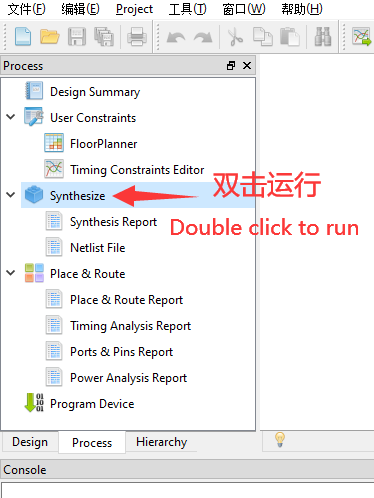### 管脚约束

PORT PIN PORT PIN PORT PIN
LCD_CLK 11 nRST 14 XTAL_IN 35
LCD_B 45 LCD_B 44 LCD_B 43
LCD_B 42 LCD_B 41 LCD_G 40
LCD_G 39 LCD_G 38 LCD_G 34
LCD_G 33 LCD_G 32 LCD_R 31
LCD_R 30 LCD_R 29 LCD_R 28
LCD_R 27 LCD_DEN 5 LCD_SYNC 46
LCD_HYNC 10

### 布局布线